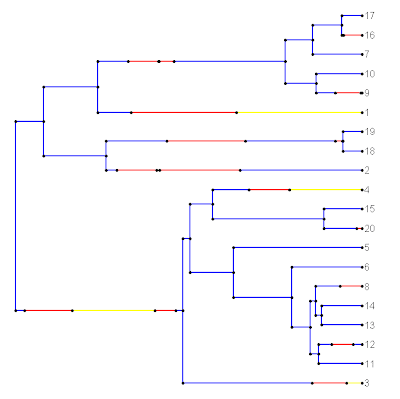## Saturday, July 9, 2011

### New plotting options in plotSimmap()

Last night I added a few new simple plotting options to the function plotSimmap(). These don't require much explanation, and include ftype (options "reg", the default, "i", "b", and "bi"); lwd, an integer the line width for edges in the tree; and pts, a logical value indicating whether or not to plot filled circles at all vertices of the tree and at state transitions.

To see how these options can be used, lets first plot using the default values. The current tree has a three state mapped character, with states 1, 2, and 3.

> cols<-c("blue","yellow","red"); names(cols)<-c(1,2,3)
> plotSimmap(mtree,cols)

The first line is to create a vector with the colors we want; and the second line plots the tree. We get:Now, let's get rid of the circles and plot the lines a little bit more thickly. (This is more like what we'd see if we used FigTree, for instance.) We can change the font to italics as well if we want:

> plotSimmap(mtree,cols,lwd=5,pts=F,ftype="i")

Now we get:Updates are in the newest version (v0.4) of this function as well in the latest alpha version of the "phytools" package (available here)